MEMO 2009 ekipno problem 6

Kvaliteta:
Avg: 0,0
Težina:
Avg: 6,0
Suppose that$ABCD$ is a cyclic quadrilateral and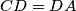$CD=DA$. Points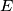$E$ and$F$ belong to the segments$AB$ and$BC$ respectively, and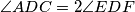$\angle ADC=2\angle EDF$. Segments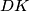$DK$ and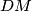$DM$ are height and median of triangle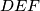$DEF$, respectively.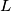$L$ is the point symmetric to$K$ with respect to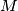$M$. Prove that the lines$DM$ and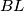$BL$ are parallel.
Izvor: Srednjoeuropska matematička olimpijada 2009, ekipno natjecanje, problem 6